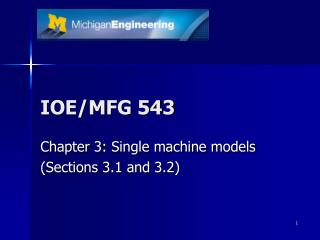DownloadDownload PresentationIOE/MFG 543

# IOE/MFG 543

Download Presentation## IOE/MFG 543

- - - - - - - - - - - - - - - - - - - - - - - - - - - E N D - - - - - - - - - - - - - - - - - - - - - - - - - - -
##### Presentation Transcript

1. IOE/MFG 543 Chapter 3: Single machine models (Sections 3.1 and 3.2)

2. Sec. 3.1: The total weighted completion time 1||S wjCj • Criteria for scheduling • Shorter jobs processed first => more jobs finish early • The higher the weight the earlier a job should finish • Weighted shortest processing time first rule (WSPT) • order the jobs in decreasing order of wj /pj • Theorem 3.1.1 • The WSPT is optimal for 1||S wjCj

3. Precedence constraints • Chains 12 ... k • r -factor (largest ratio of weight and processing times)

4. Algorithm 3.1.4 for chains • Whenever a machine is freed, schedule among the remaining chains the one with the highest r –factor • Process this chain without interruption up to and including the job l* that determines the r –factor Do example on slide 7

5. 1|| Σwj(1-e-rCj) • Weighted discounted shortest processing time first rule (WDSPT) • Order jobs in non-increasing order of wje-rpj 1-e-rpj • Gives the optimal schedule (Thm. 3.1.6) • Algorithms for chains also exist

6. Summary of other completion time models

7. Example 3.1.5 • There are 2 chains 12 34 and 567 • The weights and processing times are • Determine the schedule that minimizes the total weighted completion time

8. Section 3.2The maximum lateness • Due date related • The problem 1 || Lmax is easy • For a single machine problem with regular objective functions Cmax=Spj • Lmax=maxi{1,…,n} Li (Li =Ci-di) • Select jobs in increasing order of their due dates => earliest due date first rule (EDD)

9. Backward algorithm for1|prec|hmax • hmax=max(h1(C1),…,hn(Cn)) • The functions hi are nondecreasing • Jis the set of jobs already scheduled • Jobs in J are processed in the interval • Jcis the set of jobs still to be scheduled • J'is the set of jobs that can be scheduled (schedulable jobs)

10. Algorithm 3.2.1 for 1|prec|hmax • Set J=, Jc={1,…,n} and let J'be all jobs that have no successors • Determine Add j* to J Delete j* from Jc Modify J'to represent the new set of schedulable jobs (have no successors) • If Jc=  STOP, otherwise go to 2

11. Example 3.2.3 • Use Algorithm 3.2.1 to determine the schedule that minimizes hmax and compute the optimal value

12. Theorem 3.2.2. • Algorithm 3.2.1. yields an optimal schedule for 1 | prec | hmax • Proof:

13. Release dates 1 | rj | Lmax • Significantly harder than 1 | prec| Lmax • It may be optimal to keep the machine idle to wait for the release of a new job (not a nondelay schedule) • Theorem 3.2.4. • The problem 1 | rj | Lmax is strongly NP-hard • Reduces from 3-PARTITION

14. Branch and bound • A method of effective enumeration • Generate a node tree • Compute the objective of a feasible schedule (feasible node) • Compute lower bounds for a class of schedules (node) • The node can be eliminated if the lower bound is higher than the cost of a schedule obtained earlier

15. Branch and bound for1 | rj | Lmax • Branching • Level 0: single node and no jobs have been scheduled • Level 1: n nodes such that job j is scheduled first at node j • Level k: jobs in the first k positions have been specified

16. Branch and bound for1 | rj | Lmax (2) • Branching • At a node let J be the set of jobs that have not been scheduled • Also let t be the time when a job can start processing at the node • Only create a node for job j at the node if rj<minlJ(max(t,rl)+pl)

17. Branch and bound for1 | rj | Lmax (3) • Bounding • At each node compute the optimal value using 1 | rj , prmp| Lmax for the remaining jobs • Preemptive EDD rule is optimal for 1 | rj , prmp| Lmax • If the preemptive solution gives a non-preemptive schedule then it is feasible

18. Example 3.2.5 • Use branch and bound to determine the schedule for 1 | rj | Lmax and compute the optimal value for